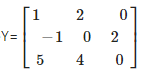# RD Sharma Solutions For Class 12 Maths Exercise 5.2 Chapter 5 Algebra of Matrices

RD Sharma Solutions for Class 12 Maths Exercise 5.2 Chapter 5 Algebra of Matrices is provided here for students to study and prepare for their board exams. Regular practice on a daily basis is the main thing that should be done by the students in the Mathematics field. Highly experienced subject experts having a vast knowledge of concepts prepare the answers, which match the understanding ability of the students.

RD Sharma Solutions for Class 12 can be used by students in order to excel in the subject by obtaining a good academic score. This exercise covers the topic addition of matrices with explanatory answers. RD Sharma Solutions for Class 12 Maths Chapter 5 Algebra of Matrices Exercise 5.2 are provided here.

## Download the PDF of RD Sharma Solutions For Class 12 Chapter 5 – Algebra of matrices Exercise 5.2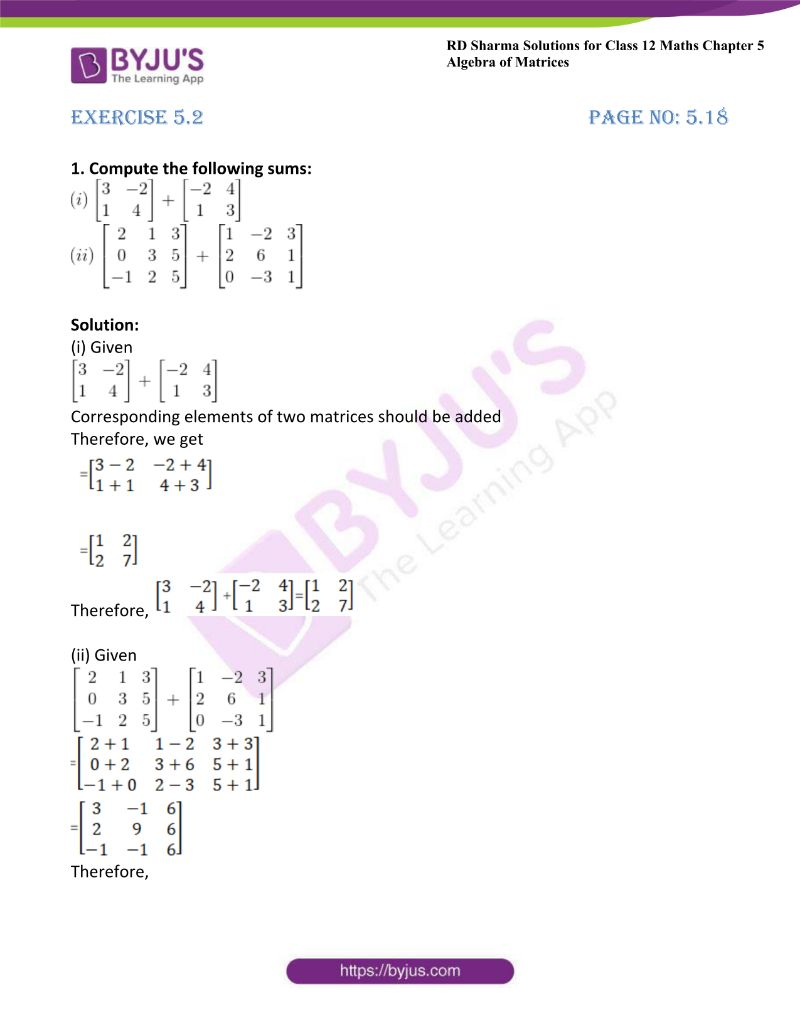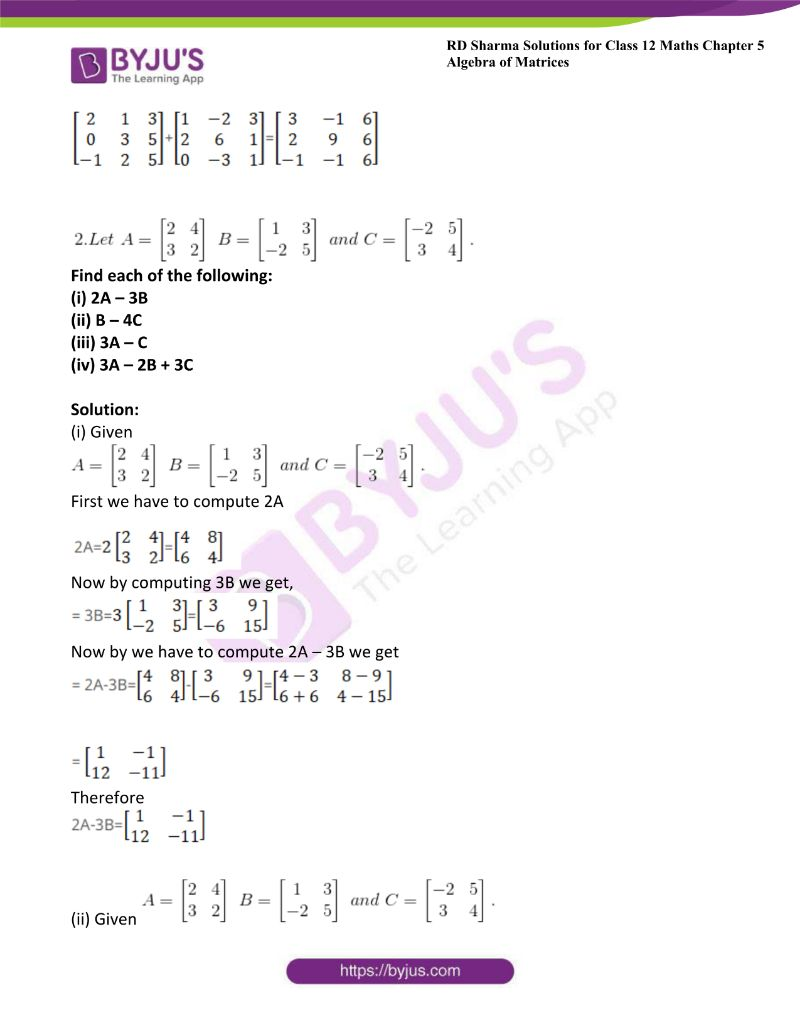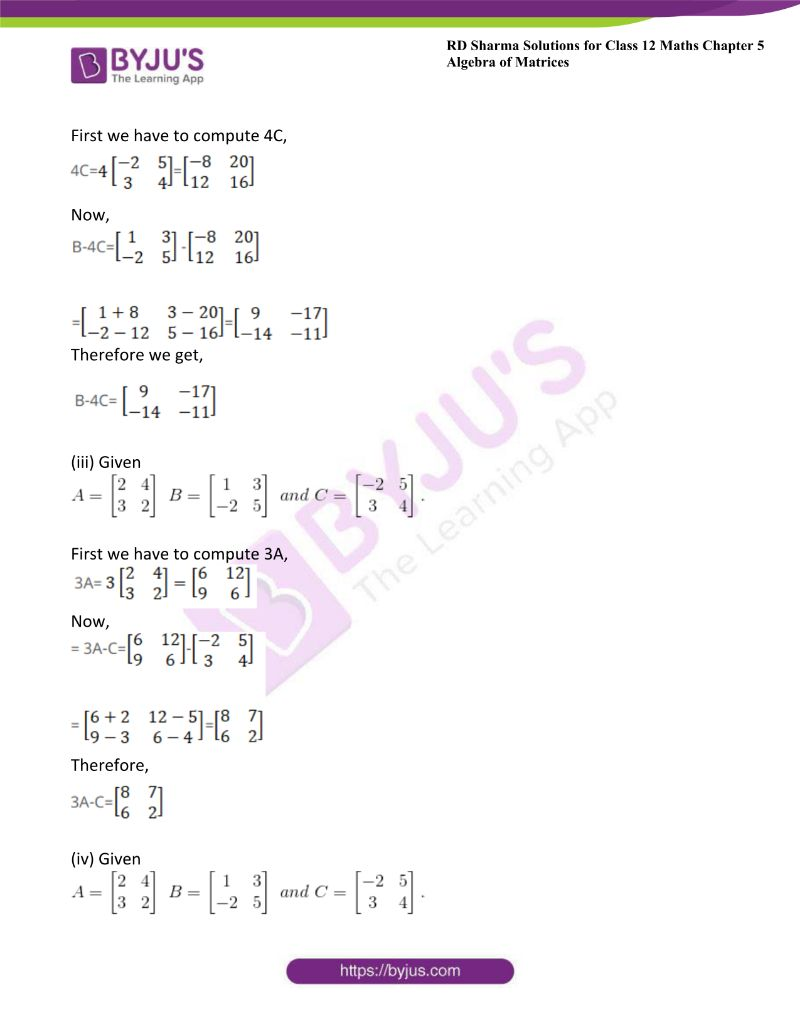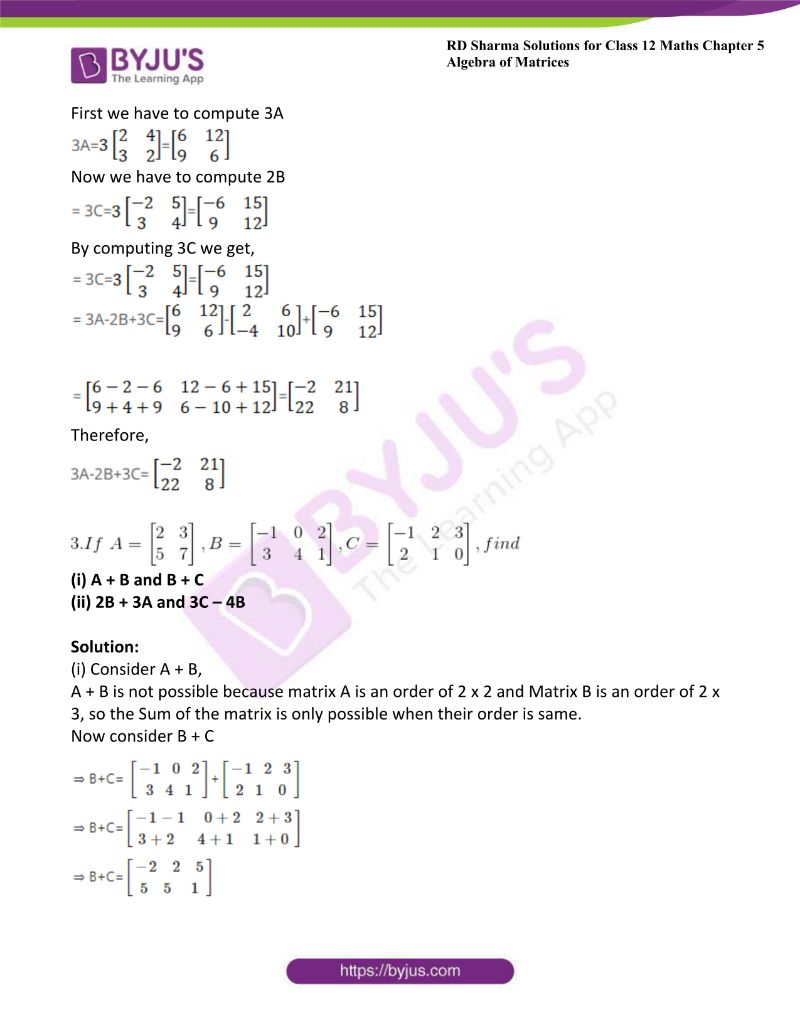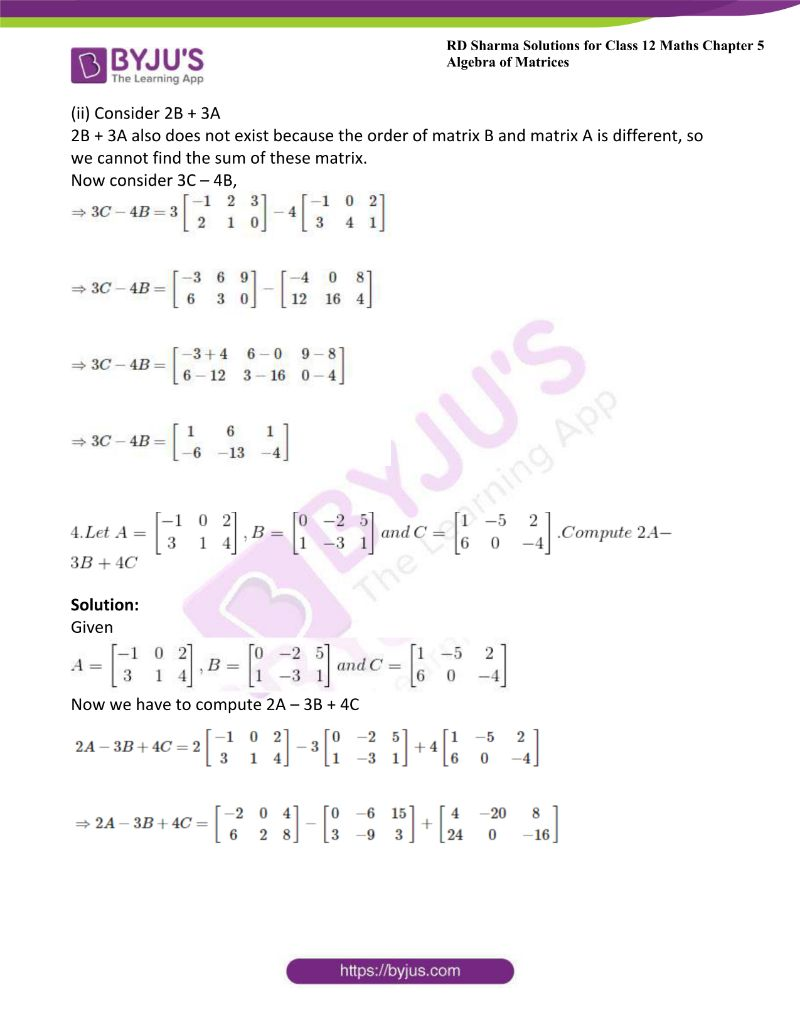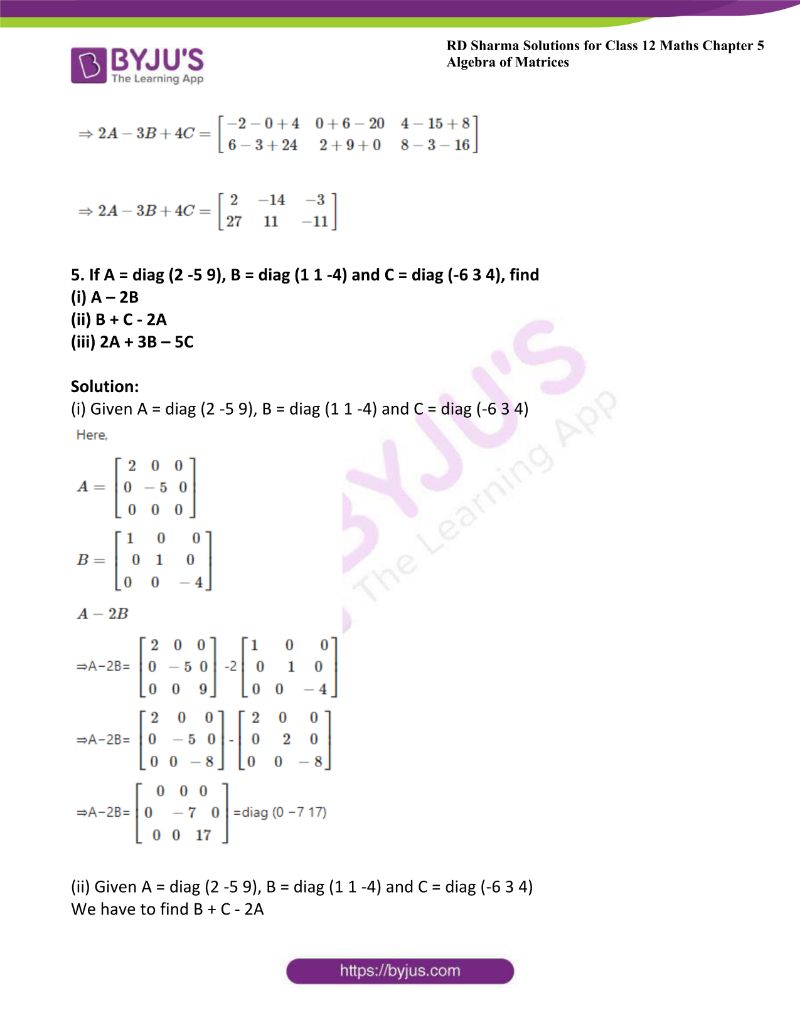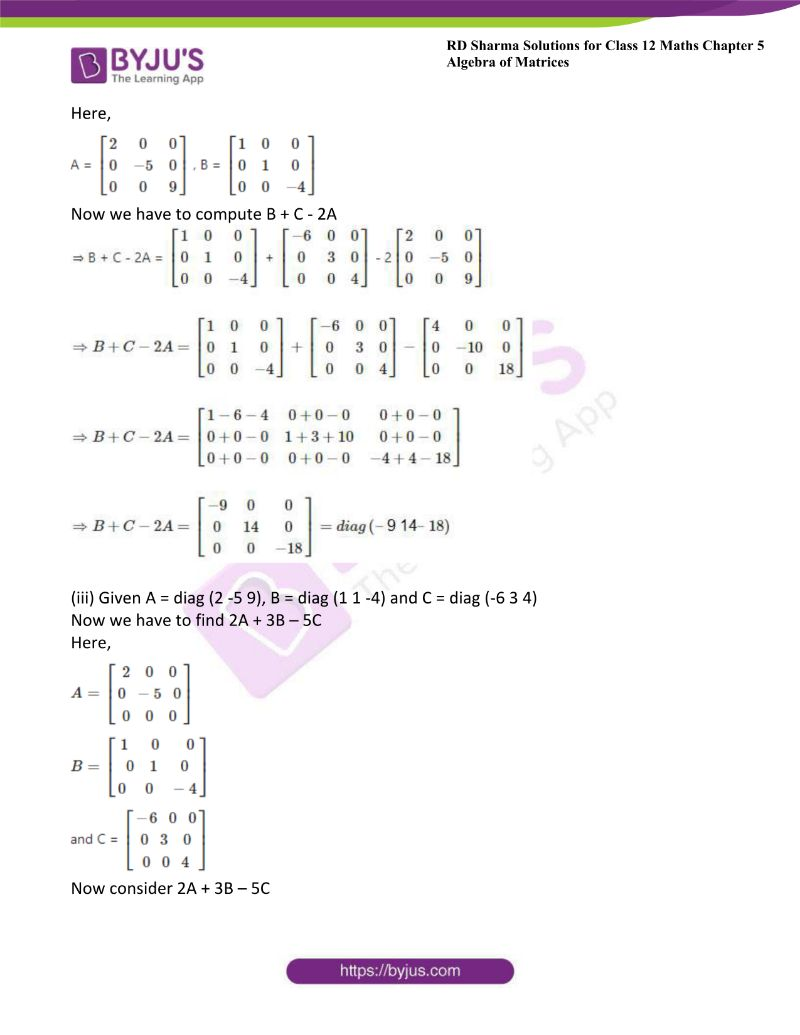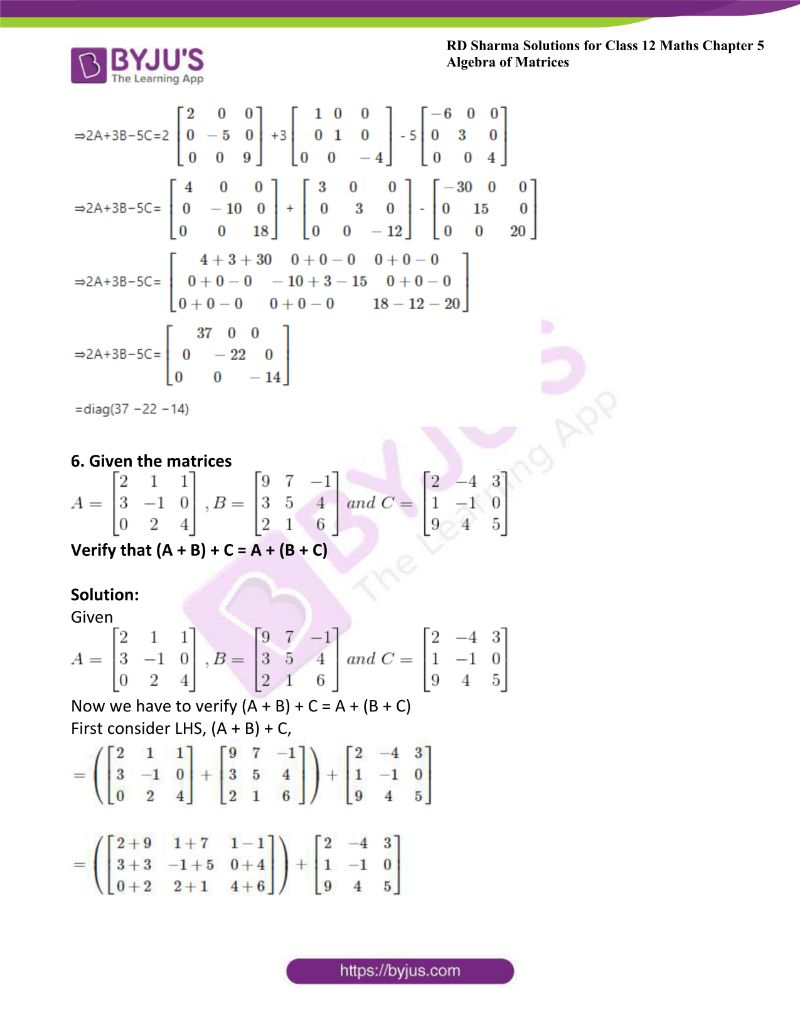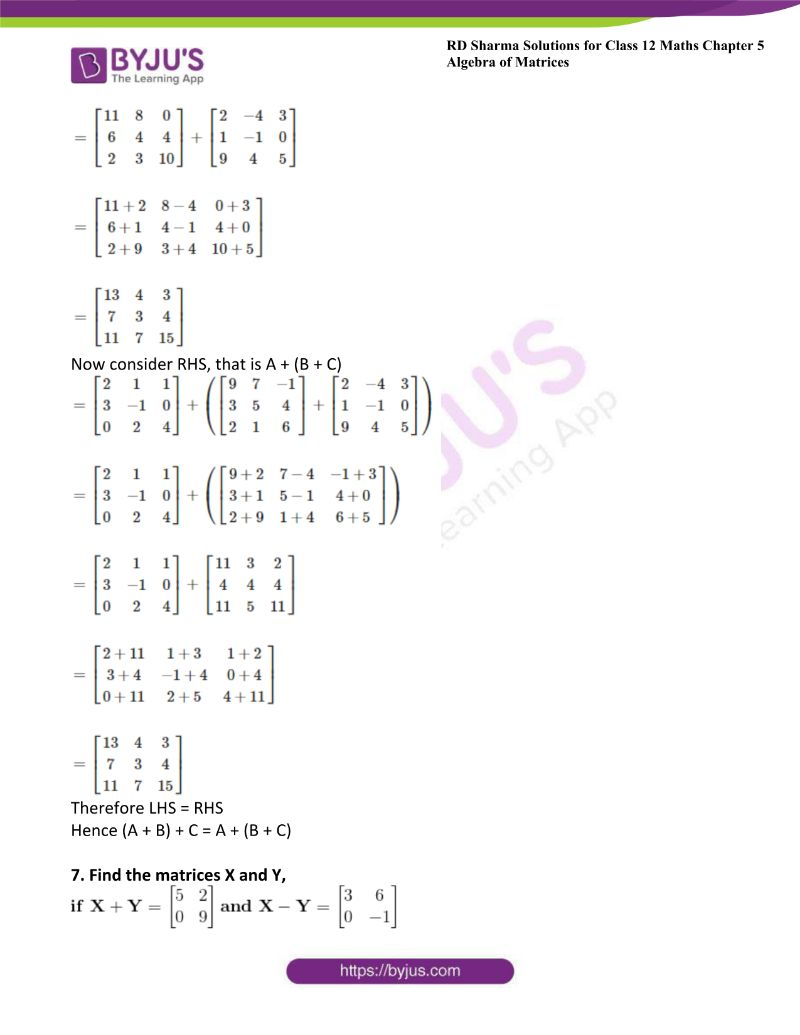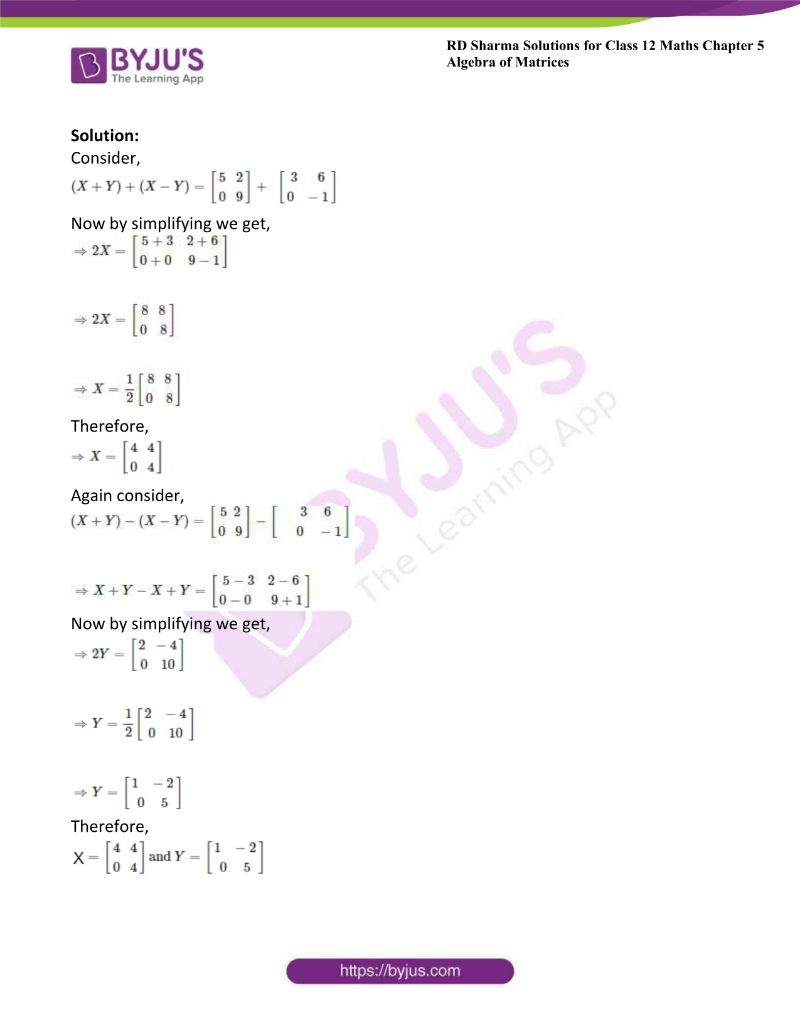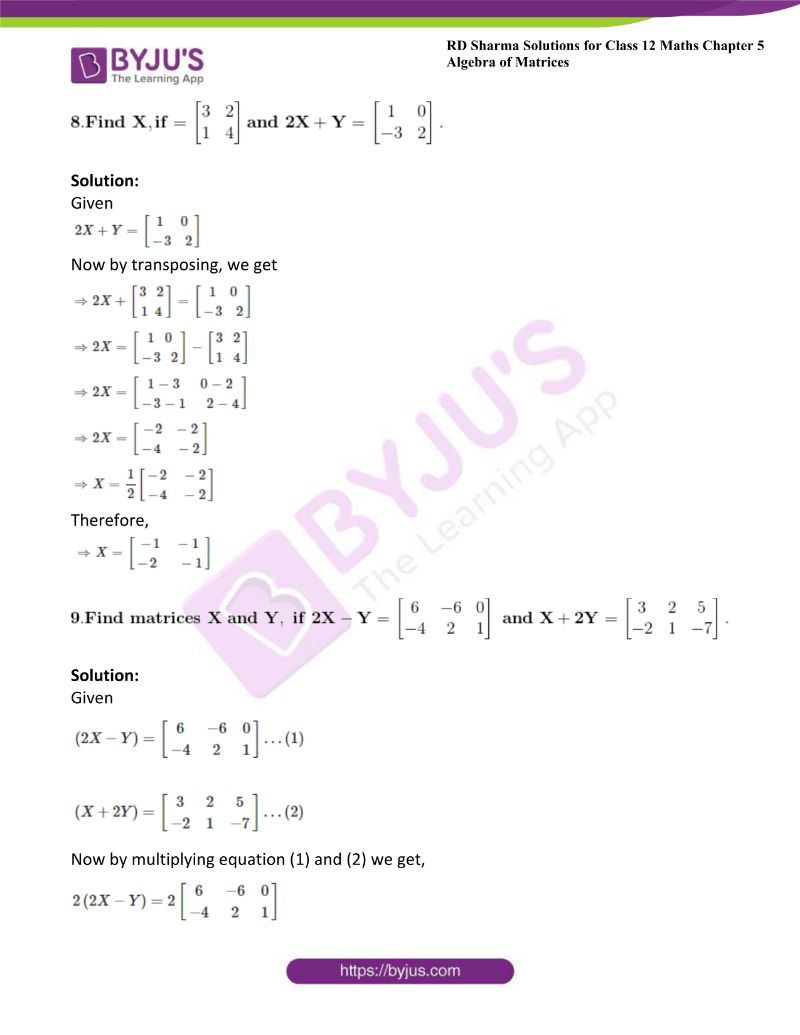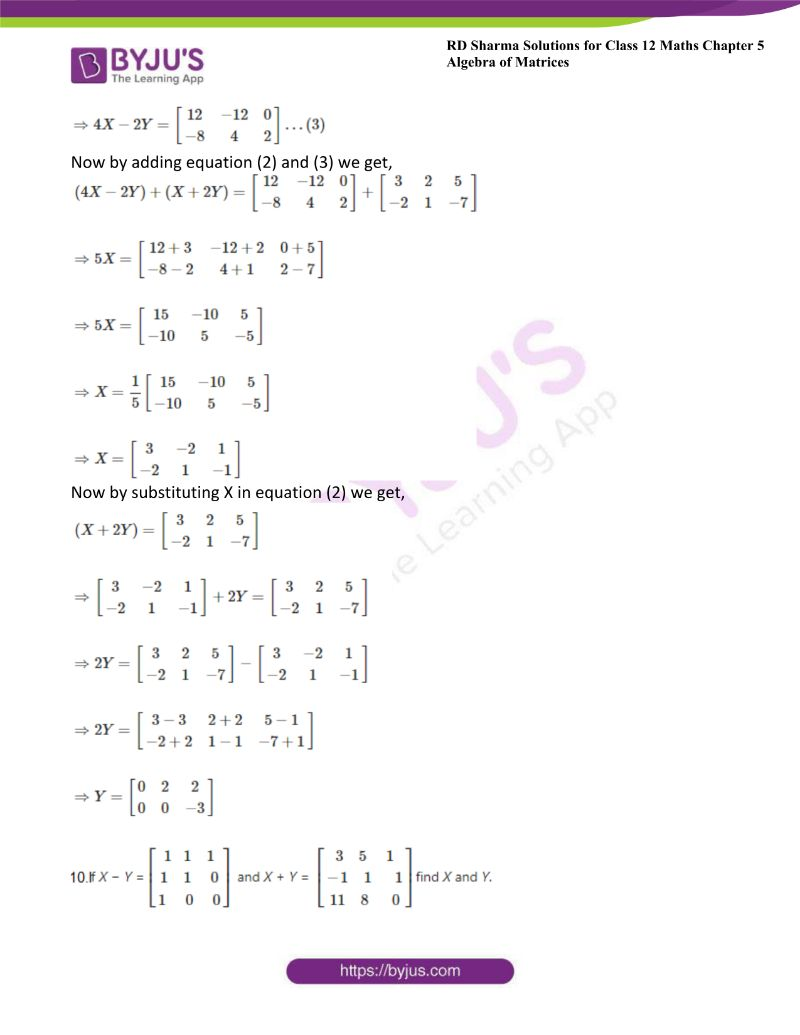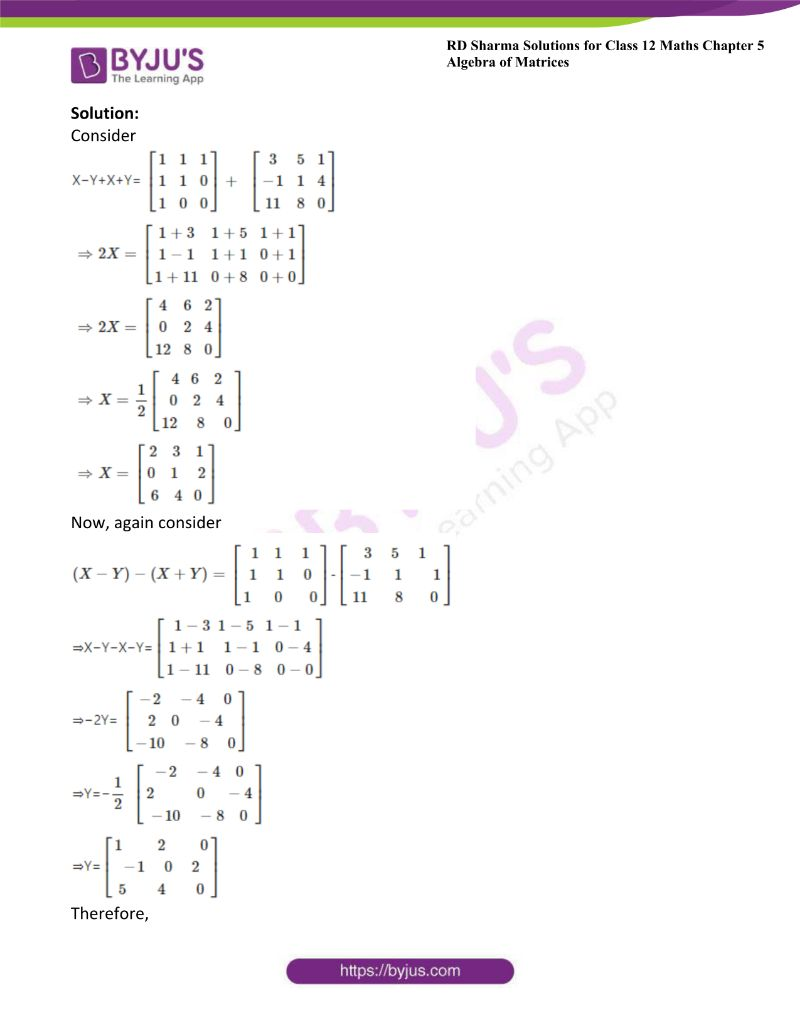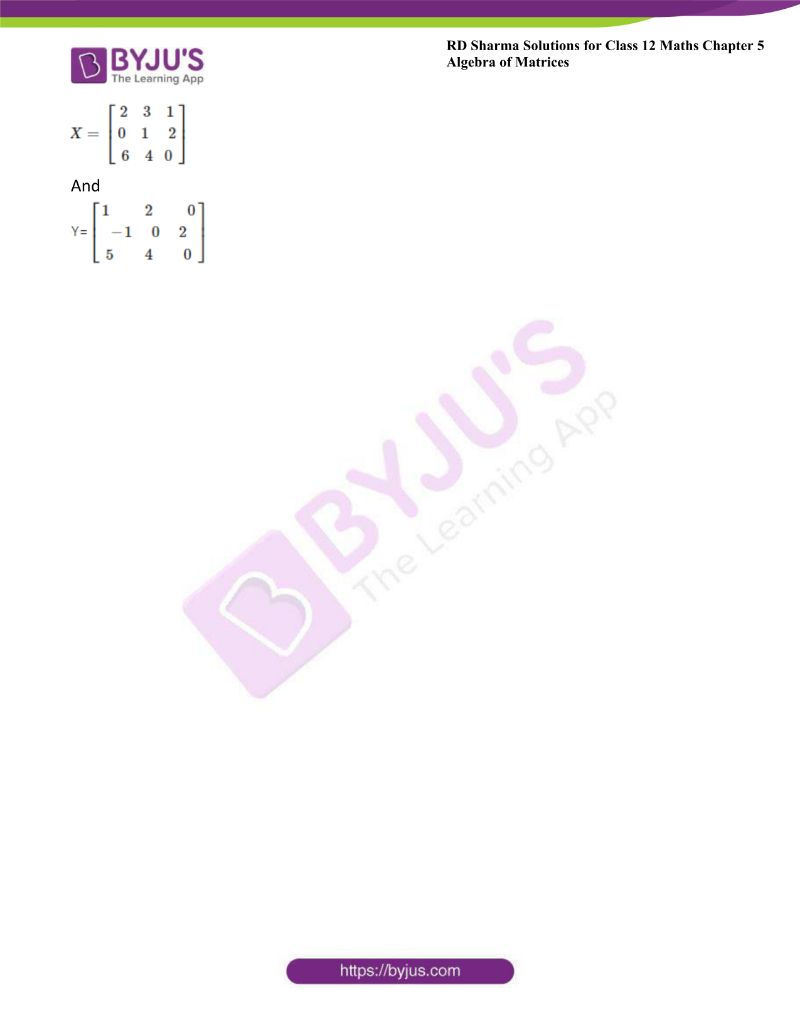### Access other exercises of RD Sharma Solutions For Class 12 Chapter 5 – Algebra of Matrices

Exercise 5.1 Solutions

Exercise 5.3 Solutions

Exercise 5.4 Solutions

Exercise 5.5 Solutions

### Access answers to Maths RD Sharma Solutions For Class 12 Chapter 5 – Algebra of Matrices Exercise 5.2

1. Compute the following sums: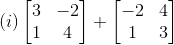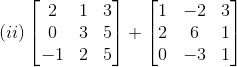Solution:

(i) GivenCorresponding elements of two matrices should be added

Therefore, we get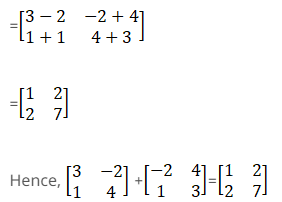Therefore,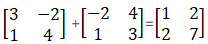(ii) Given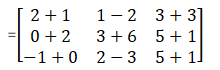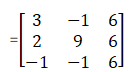Therefore,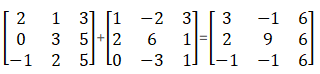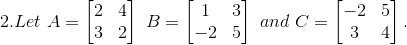Find each of the following:

(i) 2A – 3B

(ii) B – 4C

(iii) 3A – C

(iv) 3A – 2B + 3C

Solution:

(i) GivenFirst we have to compute 2A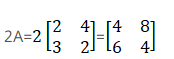Now by computing 3B we get,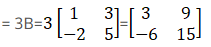Now by we have to compute 2A – 3B we get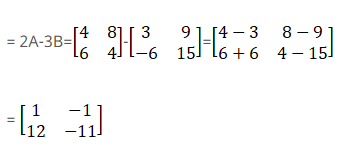Therefore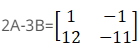(ii) GivenFirst we have to compute 4C,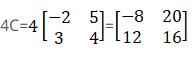Now,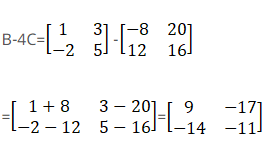Therefore we get,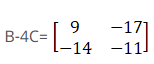(iii) GivenFirst we have to compute 3A,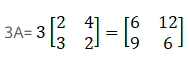Now,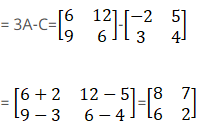Therefore,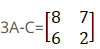(iv) GivenFirst we have to compute 3A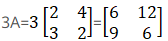Now we have to compute 2B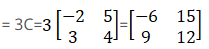By computing 3C we get,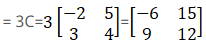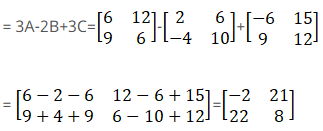Therefore,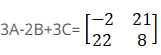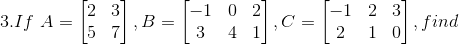(i) A + B and B + C

(ii) 2B + 3A and 3C – 4B

Solution:

(i) Consider A + B,

A + B is not possible because matrix A is an order of 2 x 2 and Matrix B is an order of 2 x 3, so the Sum of the matrix is only possible when their order is same.

Now consider B + C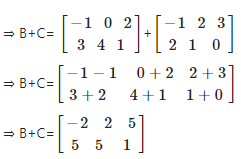(ii) Consider 2B + 3A

2B + 3A also does not exist because the order of matrix B and matrix A is different, so we cannot find the sum of these matrix.

Now consider 3C – 4B,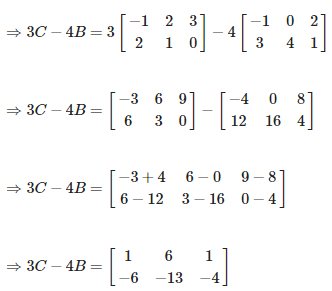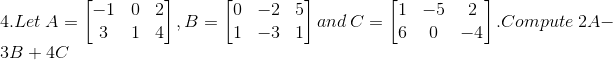Solution:

Given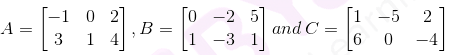Now we have to compute 2A – 3B + 4C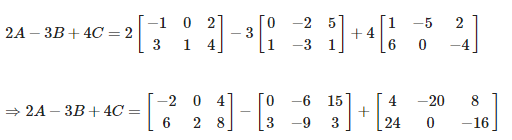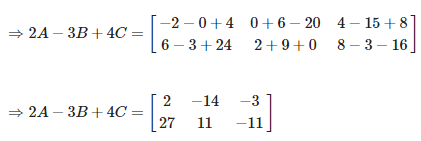5. If A = diag (2 -5 9), B = diag (1 1 -4) and C = diag (-6 3 4), find

(i) A – 2B

(ii) B + C – 2A

(iii) 2A + 3B – 5C

Solution:

(i) Given A = diag (2 -5 9), B = diag (1 1 -4) and C = diag (-6 3 4)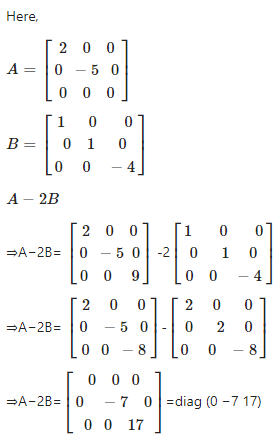(ii) Given A = diag (2 -5 9), B = diag (1 1 -4) and C = diag (-6 3 4)

We have to find B + C – 2A

Here,Now we have to compute B + C – 2A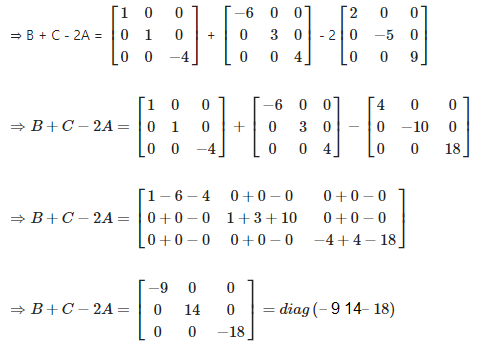(iii) Given A = diag (2 -5 9), B = diag (1 1 -4) and C = diag (-6 3 4)

Now we have to find 2A + 3B – 5C

Here,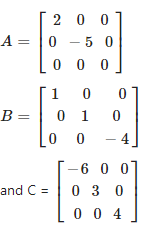Now consider 2A + 3B – 5C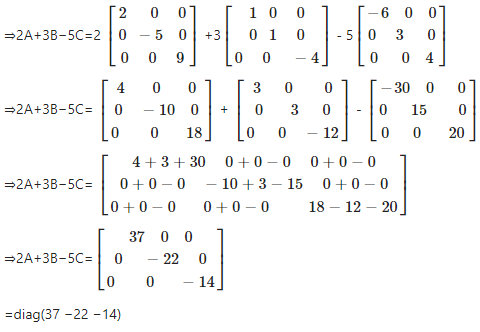6. Given the matrices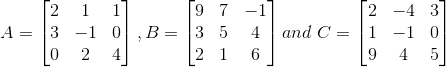Verify that (A + B) + C = A + (B + C)

Solution:

GivenNow we have to verify (A + B) + C = A + (B + C)

First consider LHS, (A + B) + C,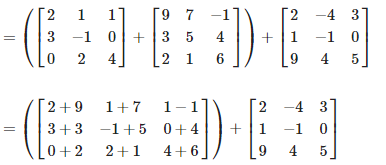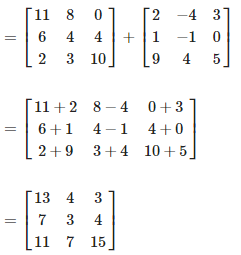Now consider RHS, that is A + (B + C)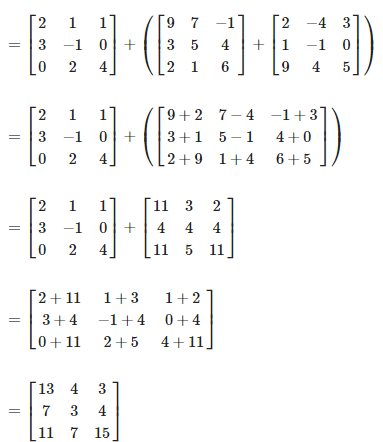Therefore LHS = RHS

Hence (A + B) + C = A + (B + C)

7. Find the matrices X and Y,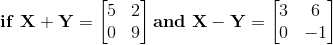Solution:

Consider,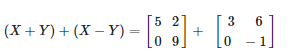Now by simplifying we get,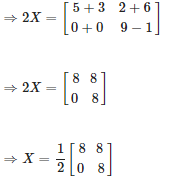Therefore,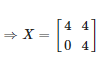Again consider,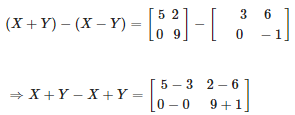Now by simplifying we get,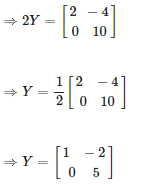Therefore,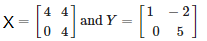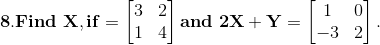Solution:

Given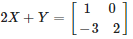Now by transposing, we get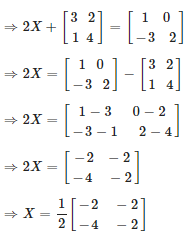Therefore,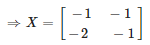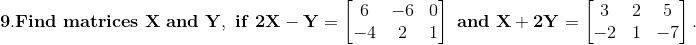Solution:

Given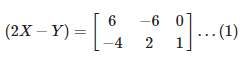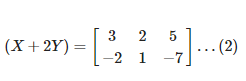Now by multiplying equation (1) and (2) we get,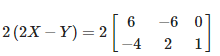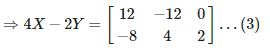Now by adding equation (2) and (3) we get,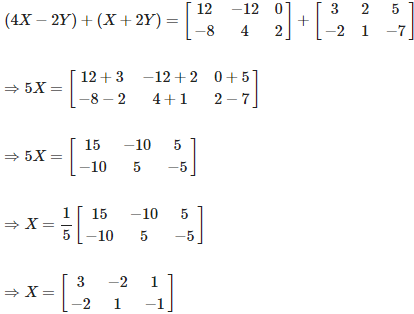Now by substituting X in equation (2) we get,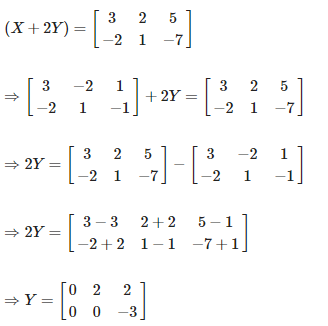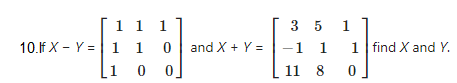Solution:

Consider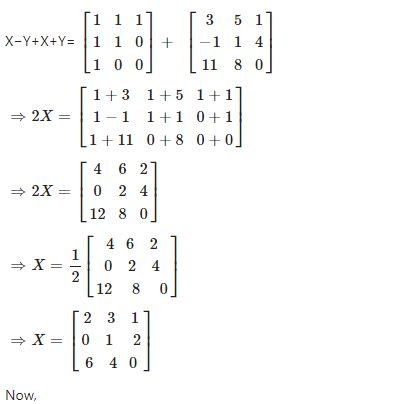Now, again consider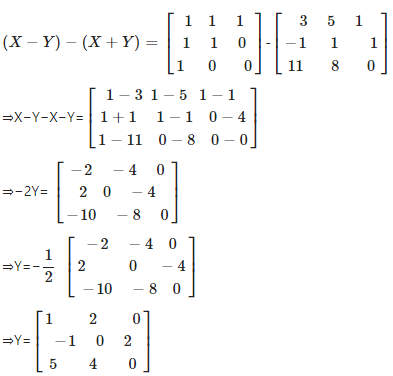Therefore,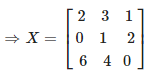And